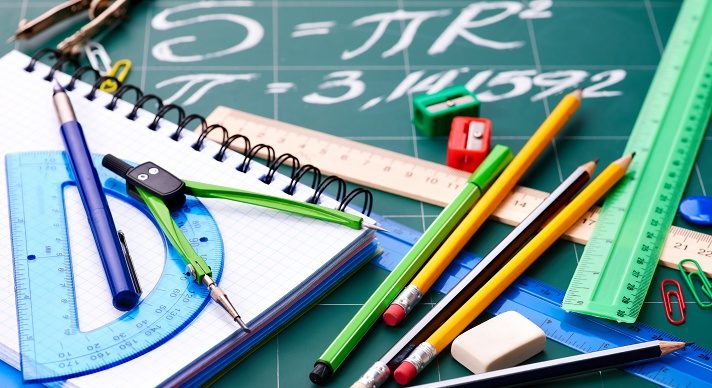# Geometry-SE### Course Overview

Designed for special education, Acellus Geometry-SE teaches students the basic principles of Euclidian Geometry including points, lines, angles, surfaces, and solids. Geometry-SE is recommended for students in grades 9-12 who have completed Acellus Algebra-SE, to ensure that these students have a strong foundation upon which to build. Course topics include:
• Points, Segments, Length
• Pythagorean Theorem
• Rays, Angles, Planes
• Congruency
• Logic Reasoning (truth tables)
• Transformations
• Postulates and Theorems of Geometry
• Geometric Proofs
• Complement and Supplement
• Properties of Triangles
• Special Triangles (30-60-90 and 45-45-90)
• Polygons
• Polyhedra
• Euler’s Formula
• Similar Figures
• Trigonometric Ratios
• Properties of Circles
• Arc, Arc Length, Chords, and Sectors
• Surface Area
• Volume
This course is based on NCTM standards. It is taught by award-winning Acellus Master Teacher, Patrick Mara.This course was developed by the International Academy of Science. Learn More

### Scope and Sequence

Unit 1 – Measure This unit covers the pythagorean theorem, rays, angles, and planes, measuring angles and perpendiculars, and congruency in size and shape. Unit 2 – Logic This unit discusses inductive and deductive reasoning, if-then statements, truth tables, postulates, and proofs. Unit 3 – Transformations This unit covers reflections, rotations, translations, dilations, tessellations, and symmetry. Unit 4 – Congruent Angles This unit discusses the angle-addition and corresponding-angle postulates, complement and supplement, vertical angles, angle bisectors, transversals, and alternate interior and corresponding angles. Unit 5 – Triangles - Part A This unit covers triangle classification, the 180 Degree Theorem, exterior angles, and congruency of triangles. Also covered are SSS and SAS, as well as ASA and SAA. Unit 6 – Area & Pythagorean Theorem This unit discusses area versus perimeter, polygons, and 30-60-90 and 45-45-90 triangles. Unit 7 – Polygons This unit discusses quadrilaterals, parallelograms, rhombi, rectangles, squares, polygons, regular polygons, and polyhedra. Unit 8 – Similarity This unit defines similarity and discusses the perimeter and area of similar figures, as well as AA, SSS, and SAS. Unit 9 – Circles This unit covers radius, diameter, and chord, as well as tangent lines and formulas for circumference and area of circles. Unit 10 – Surface Area This unit includes surface area of prisms, cylinders, and spheres. Unit 11 – Volume This unit discusses the volume of prisms, cylinders, and spheres.